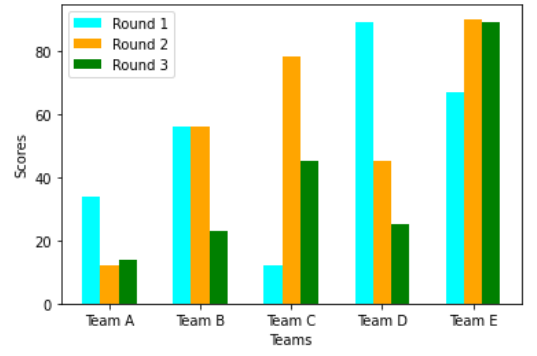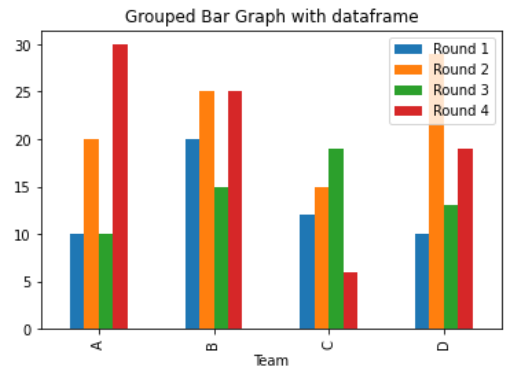Related Articles
Create a grouped bar plot in Matplotlib
• Last Updated : 17 Dec, 2020

In this article, we will learn how to Create a grouped bar plot in Matplotlib. Let’s discuss some concepts :

• Matplotlib is a tremendous visualization library in Python for 2D plots of arrays. Matplotlib may be a multi-platform data visualization library built on NumPy arrays and designed to figure with the broader SciPy stack. It had been introduced by John Hunter within the year 2002.
• A bar plot or bar graph may be a graph that represents the category of knowledge with rectangular bars with lengths and heights that’s proportional to the values which they represent. The bar plots are often plotted horizontally or vertically.
• A bar chart is a great way to compare categorical data across one or two dimensions. More often than not, it’s more interesting to compare values across two dimensions and for that, a grouped bar chart is needed.

Approach:

1. Import Library (Matplotlib)
2. Import / create data.
3. Plot the bars in the grouped manner.

Example 1: (Simple grouped bar plot)

## Python3

 `# importing package``import` `matplotlib.pyplot as plt``import` `numpy as np`` ` `# create data``x ``=` `np.arange(``5``)``y1 ``=` `[``34``, ``56``, ``12``, ``89``, ``67``]``y2 ``=` `[``12``, ``56``, ``78``, ``45``, ``90``]``width ``=` `0.40`` ` `# plot data in grouped manner of bar type``plt.bar(x``-``0.2``, y1, width)``plt.bar(x``+``0.2``, y2, width)`

Output :Example 2: (Grouped bar chart with more than 2 data)

## Python3

 `# importing package``import` `matplotlib.pyplot as plt``import` `numpy as np`` ` `# create data``x ``=` `np.arange(``5``)``y1 ``=` `[``34``, ``56``, ``12``, ``89``, ``67``]``y2 ``=` `[``12``, ``56``, ``78``, ``45``, ``90``]``y3 ``=` `[``14``, ``23``, ``45``, ``25``, ``89``]``width ``=` `0.2`` ` `# plot data in grouped manner of bar type``plt.bar(x``-``0.2``, y1, width, color``=``'cyan'``)``plt.bar(x, y2, width, color``=``'orange'``)``plt.bar(x``+``0.2``, y3, width, color``=``'green'``)``plt.xticks(x, [``'Team A'``, ``'Team B'``, ``'Team C'``, ``'Team D'``, ``'Team E'``])``plt.xlabel(``"Teams"``)``plt.ylabel(``"Scores"``)``plt.legend([``"Round 1"``, ``"Round 2"``, ``"Round 3"``])``plt.show()`

Output :Example 3: (Grouped Bar chart using dataframe plot)

## Python3

 `# importing package``import` `matplotlib.pyplot as plt``import` `pandas as pd`` ` `# create data``df ``=` `pd.DataFrame([[``'A'``, ``10``, ``20``, ``10``, ``30``], [``'B'``, ``20``, ``25``, ``15``, ``25``], [``'C'``, ``12``, ``15``, ``19``, ``6``],``                   ``[``'D'``, ``10``, ``29``, ``13``, ``19``]],``                  ``columns``=``[``'Team'``, ``'Round 1'``, ``'Round 2'``, ``'Round 3'``, ``'Round 4'``])``# view data``print``(df)`` ` `# plot grouped bar chart``df.plot(x``=``'Team'``,``        ``kind``=``'bar'``,``        ``stacked``=``False``,``        ``title``=``'Grouped Bar Graph with dataframe'``)`

Output :Attention geek! Strengthen your foundations with the Python Programming Foundation Course and learn the basics.

To begin with, your interview preparations Enhance your Data Structures concepts with the Python DS Course.

My Personal Notes arrow_drop_up